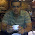## Thursday, March 14, 2013

### How to remove leading zeros in a string in Linux?

How to remove leading zeros in a variable or a string?

Let us consider a variable "x" which has the below value :
```\$ x=0010
\$ echo \$x
0010```
1. Using typeset command of ksh:
```\$ typeset -LZ x
\$ x=0010
\$ echo \$x
10```
The variable x is declared using the typeset command. The -Z option of typeset will strip the leading zeros present when used along with -L option.

2. Using sed command:
```\$ echo \$x | sed 's/^0*//'
10```
The expression '^0*' will search for a sequence of 0's in the beginning and delete them.

3. Using awk :
```\$ echo \$x | awk '{sub(/^0*/,"");}1'
10```
Using the sub function of awk, explanation same as sed solution.

4. awk with printf:
```\$ echo \$x| awk '{printf "%d\n",\$0;}'
10```
Using printf, use the integer format specifier %d, the leading zeros get stripped off automatically.

5. awk using int:
```\$ echo \$x | awk '{\$0=int(\$0)}1'
10```
The int function converts a expression into a integer.

6. Perl using regex:
```\$ echo \$x | perl -pe 's/^0*//;'
10```

7. Perl with printf:
```\$ echo \$x | perl -ne 'printf "%d\n",\$_;'
10```

8. Perl with int:
```\$ echo \$x | perl -pe '\$_=int;'
10```1.Nice article dude . But I did not understand the significance of 1 from the followed line

\$ echo \$x | awk '{sub(/^0*/,"");}1'

Would you please let me know why do we need to provide 1
at the end.

1.1 is to print every line.

2.Instead of 1 , I tried giving 2..10 numbers , all of them worked perfectly as
we gave 1 earlier.So my question is , Can we use any number ? or Is there any
special difference that you know ?

3.Any non-zero number will do. A non-zero number evaluates to true and awk prints a line when true.

2.using arithmetic espansion:
\$ echo \$((10#\$x))
10